# Preventing output polarity inversion in high-speed amplifiers

Many control loop applications require that you avoid unexpected polarity inversion with respect to input. This is because the control loop’s response can cause system instability if the output of one stage changes polarity in an unexpected manner. In this post, I’ll look into the issue and suggest a simple method to avoid it in a circuit.

What is polarity inversion, and what causes it?
Let’s consider an example of a signal chain with a rail-to-rail output (RRO) amplifier powered by +-5V, followed by another driving amplifier with a bipolar input stage running at +-5V supplies and gain >1. In this case, the RRO amplifier saturates at output close to +/-5V, which will feed into the next amplifier. The second amplifier is expected to saturate as well, once the output reaches close to +/-5V. However, in many amplifiers, if the input is outside of the specified input common mode range, the output actually switches to the negative rail. .

For example, the THS4031, a 100-MHz, low-noise voltage-feedback amplifier, exhibits the above behavior and includes the NPN (negative positive negative) input stage used in many high-speed, low-noise amplifiers.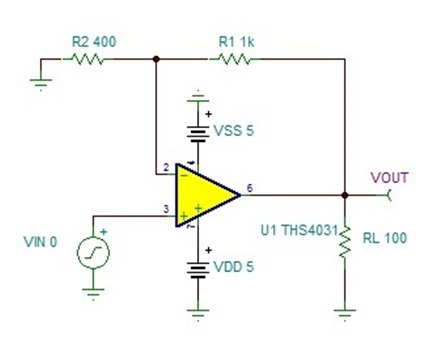Figure 1: Example circuit

The circuit in Figure 1 showed the following behavior when I ramped the input signal up to the positive rail: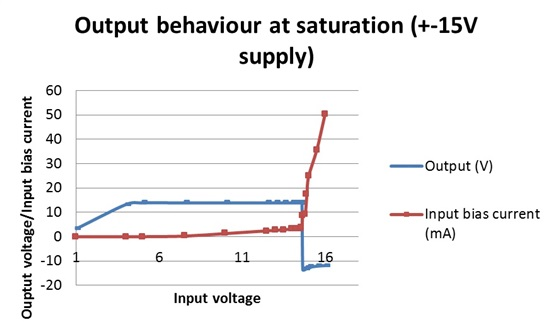Figure 2: Output v/s input at +-15V supply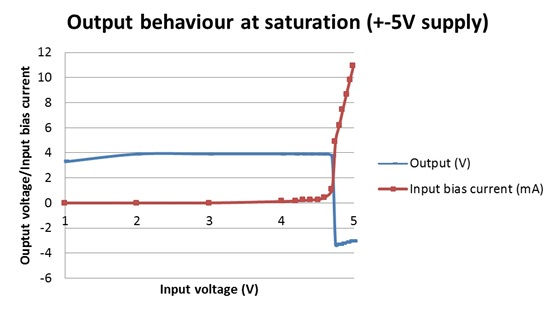Figure 3: Output v/s input at +-5V

As shown in Figures 2 and 3, the output dips below the saturation voltage when the input common mode increases. This behavior occurs as the input approaches the supply rails, where the output finally clamps at the negative saturation level. Note that the bias current also shoots up as the input approaches the supply rail. In this case, the output appears to reverse polarity when the input bias current exceeds 4mA.

Preventing output polarity inversion
You can prevent polarity inversion by using a current limit resistor at the amplifier input, as shown in Figure 4. This will limit the input bias current as the input signal approaches the positive rail and avoids any effect on the internal current bias sources.

Figure 4: Adding a current limit resistor at input

I tested the setup of this circuit to verify the absence of unexpected polarity inversion at the output, as shown in Figures 5 and 6: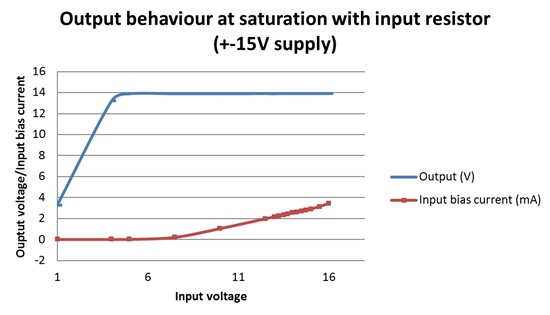Figure 5: Output v/s input with current limit resistor (+-15V)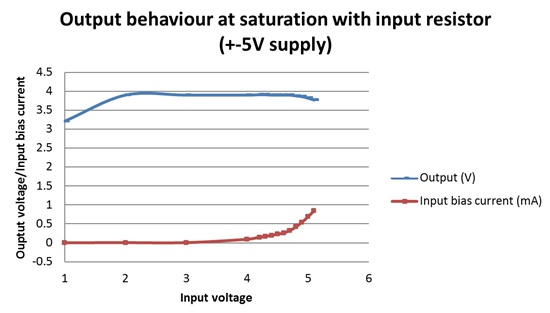Figure 6: Output v/s input with current limit resistor (+-5V)

The larger input signal positive swing will need higher resistance to limit the input bias current when the input exceeds the common mode compliance range. This will ensure that the current through the forward-biased diode on the input transistor does not exceed the biasing current source flow occurring in the opposite direction.

The resistance will have a small effect on the output noise and bandwidth (due to input capacitance of amplifier). Use this equation to calculate increase in the output voltage noise:

Eno= (noise gain)*(Current limit resistance)*(Input Current noise of the amplifier)+ Ern(resistor noise)*Noise gain.

Polarity inversion is most commonly caused by the effect of increased input bias current on an op amp’s internal current sources. Most op amp families with a PNP (positive negative positive) input stage  can inherently accept a negative-rail input, so it’s rare for those devices to exhibit this behavior at the negative rail input. Newer high-speed op amps with an NPN input stage resolve the polarity inversion issue internally. The voltage for polarity inversion is much closer to the rail in those cases. For example,  the OPA842 is a wideband, low distortion, unity gain stable voltage feedback op amp, that doesn’t show this behavior until the input reaches within .15V from the positive rail (without current limit resistor in the circuit).

Adding a current limiting resistor at the input can prevent polarity inversion issues when the input swing is close to supply rail.

If you have any questions about how to prevent output polarity in high- speed amplifiers, feel free to comment below.

I would like to acknowledge Samir Cherian and Amit Goyal from the high-speed amplifiers applications team for their support in writing this post.